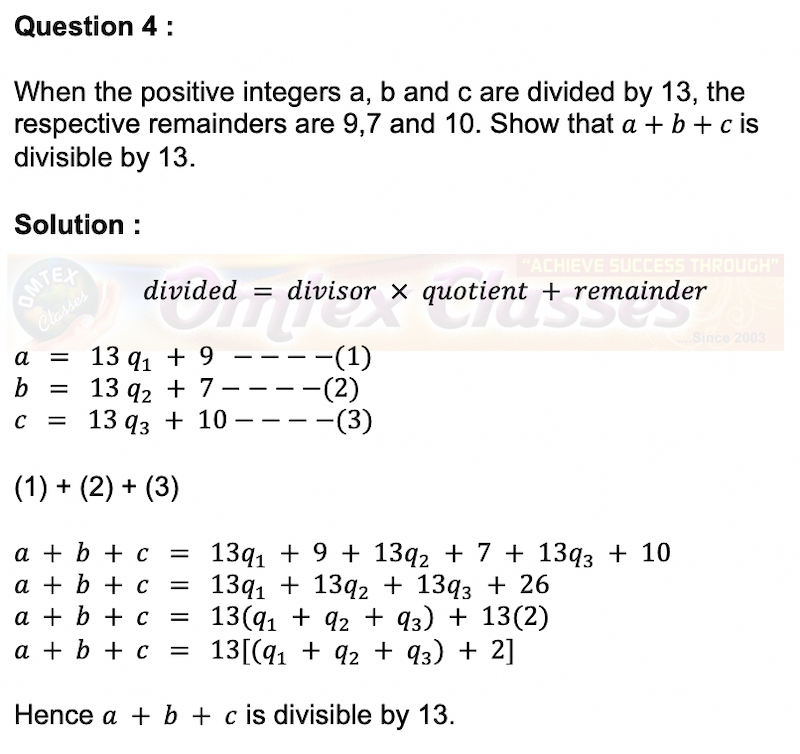### When the positive integers a, b and c are divided by 13, the respective remainders are 9,7 and 10. Show that a + b + c is divisible by 13.

SSLC 10TH STANDARD Tamil Nadu NEW SYLLABUS. 2019 - 2020.

CHAPTER 2: NUMBERS AND SEQUENCES

Exercise: 2.1

Question 4 :

When the positive integers a, b and c are divided by 13, the respective remainders are 9,7 and 10. Show that a + b + c is divisible by 13.When the positive integers a, b and c are divided by 13, the respective remainders are 9,7 and 10. Show that a + b + c is divisible by 13.